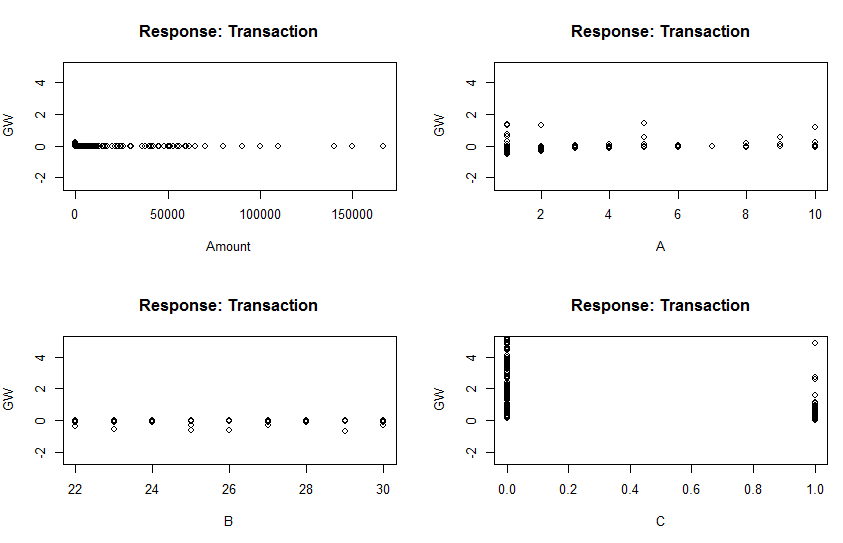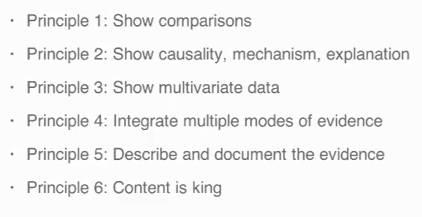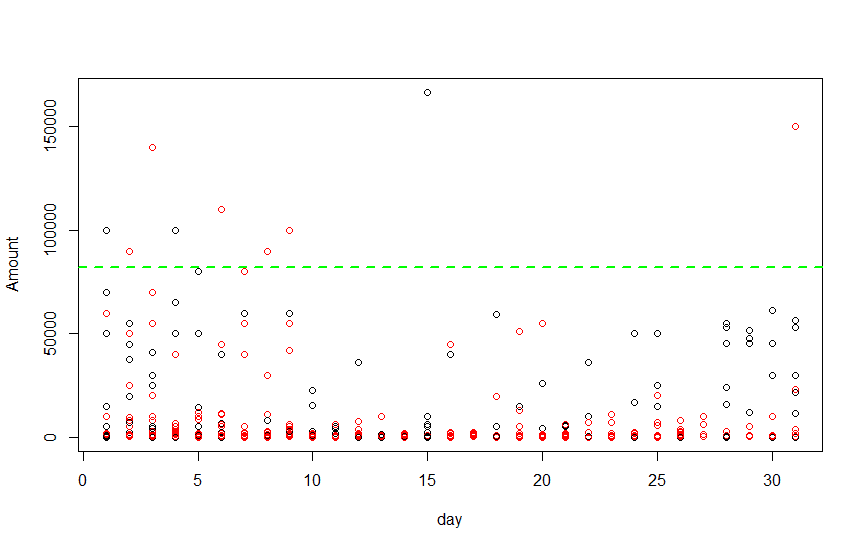## Principles of Data Analysis

Principle 1 -
- Always try to find a hypothesis which can be compared with other hypothesis. So point comes here is "compared to what ? ".

Principle 2 -
- Needs to justify the cause of framework we derive

Principle 3 -
-Show Multivariate data or more that one dimension of data , to precisely justify your  point . As data always speak and more and more data with proper representation will prove that point.Multivariate Data Representation

So this kind of graphical representation speak about generalized weight of each of the feature parameter in neural network, so multivariate data representation does precisely show the representation.

Principle 4 -
- Integration of Evidence, It means always try to show almost all dimension of data not just limited. Add words , numbers , images or almost everything you have to show the data .
So main idea is its you who drive the data to represent, not the tool which just takes the data and plot something random.

Principle 5 -
-Sources where the data came from , so kind of evidence of your plot.Main Principles of Exploratory Data Analysis

### Why Exploratory Data Analysis

• Because they can be made easily and  faster
• Can help for personal understanding
• Explain how data look like which is the main important for any Data
• Look and Feel is NOT the primary concern about exploratory data analysis

### Example of Exploratory Data Analysis

I have my Bank account details and I needed to find out is there any time when I crossed more than 80000 from my Bank account transaction in terms of Debit .

First I'll be working on 1-Dimensional Plots like Box , Hist & Bar

So now I am assuming or trying to show my data as Categorical Variable.

DataSet

So my data looks like above. First I wanted to plot a Boxplot on my Amount data to check the density of data with median and mean

`````` data <- read.csv("D:/tmp/mlclass-ex1-005/mlclass-ex3-005/R-Studio/account.csv")
#Replace the comma from Amount
data\$Amount <- as.numeric(gsub(",", "", gsub("", "", data\$Amount)))
``````

`````` #Plotting the Box Plot
boxplot(data\$Amount , col = "yellow")
#This corresponds that almost majority of my data is below 8000 , very less population around 80000
abline( h = 80000) #h = horizental
``````

Well I realized that its useless for me as I am not even able to derive anything from this plot.

So now I decided to go ahead to some better way like to draw a histogram with rug and found out -

`````` #Plotting Histogram of the data to get more details about the data
hist(data\$Amount , col = "light green" )
#rug shows exactly where the points are
rug(data\$Amount)
#rug shows that bulk of the data is in between 25000 itself
``````

Yeah this seems to better but I can make it more better by finding Median and Mean over that same graph and bring some more break points-

`````` #Adding breaks add more number of plots or break the graphs in smaller parts
hist(data\$Amount , col = "light blue" ,breaks = 20)
abline( v = 85000, lwd = 4) #v = vertical
#Since hist doesn;t show median so that as well can be added here
abline( v = median(data\$Amount) , col = "red" , lwd = 4)
``````

Great , now I have something to derive on my Data.

But how about trying the same with other feature like Transaction as I have binary value of it ie 1 for Debit or 0 for Credit.

Box Plot - a horrible mistake

Histogram , well it gives something but its not meant for this-

And finally here we find barplot is the best suit which actually can gives us the frequency of 0 or 1  , we can derive the occurrences on my credit account which is extremely poor . :(

`````` #Trying to Plot histogram of Transaction by changing to numeric
data\$Transaction <- as.numeric(data\$Transaction=="Dr.")
#You won't get any details from Box Plot of Transaction because you have just 2 entries
boxplot(data\$Transaction , col = "yellow")
#Here we can derive the frequency of 0 and 1
hist(data\$Transaction , col = "light green")
#Bar plot will be best suit for finding the majority of Debit and Credit from your account
barplot(table(data\$Transaction) , col = "light blue" , main = "Ration of Credit vs Debit ")
``````

Now to work mainly on 2-Dimensional plots like Scatter-plot

Well Here I just wanted to find what is the density of Debit and Credit for my account Transaction and I was surprised to see its "Credit"

For the same purpose , Histogram Representation where its more clearedAmount vs Days spending and Trying to find Debit or Credit on top of it

`````` data <- read.csv("D:/tmp/mlclass-ex1-005/mlclass-ex3-005/R-Studio/account.csv")
#Replace the comma from Amount
data\$Amount <- as.numeric(gsub(",", "", gsub("", "", data\$Amount)))
#Plotting 2-Dimensional Using Box Plot
#Trying plot Amounts based on Credit or Debit
boxplot(Amount ~ Transaction , data = data , col = "red")
#Credit is More than Debit , hows that possible , that is because I am comparaing
# based on the amount not with frequency , so amount speaks a total different chapter
#Histogram representation for the same purpose
hist(subset(data,Transaction == "Dr.")\$Amount, col = "green" , breaks = 20)
hist(subset(data,Transaction == "Cr.")\$Amount, col = "green" , breaks = 20)
#data\$Transaction <- as.numeric(data\$Transaction=="Dr.")
#ScatterPlot
#Split Date in Day Month & year
data\$Transaction.Date <- as.Date(data\$Transaction.Date, format="%d/%m/%Y")
month = as.numeric(format(data\$Transaction.Date, format = "%m"))
day = as.numeric(format(data\$Transaction.Date, format = "%d"))
year = as.numeric(format(data\$Transaction.Date, format = "%Y"))
#Shows the Amount transaction on Each day , and col sepeartes the value based on Dr. or Cr. type
with(data,plot(day,Amount, col = Transaction)) #col will take Dr. as color red
abline( h = 82000, lwd = 2, lty = 2, col = "green")
#points(data\$Amount,data\$Transaction == "Dr.",col = "blue")
#points(data\$Amount,data\$Transaction == "Cr.",col = "red")
#Seperate the data based on year
#with(data,plot(day,Amount, col = Year))
#MULTIPLE SCATTERPLOT like I Did in Histogram
s1 <- subset(data,Transaction == "Dr.")
s2 <- subset(data,Transaction == "Cr.")
with(s1,plot(c(1:nrow(s1)),Amount,main = "Debit"))
#day is not equal to size of x , so we need to find
with(s2,plot(c(1:nrow(s2)),Amount,main = "Credit"))
``````

So whenever the data comes, proper basic visualization is going to solve most of the trouble and that is what we did and that is called Exploratory Data Analysis.
We got the preview of data , and almost tried to follow the principles... is it .. have I actually followed all the principles....... :)

So its a kind of quick and dirty approach to summarize the data and it just ease our job to decide the model and strategy for the next step.